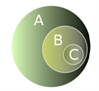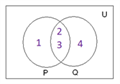# Types Of Sets

Sets are one of the most important topics in the CAT quantitative aptitude section. The questions related to sets can be easily solved and are less complicated. Know about the types of sets here with detailed explanations.

### Different Types of Sets:

#### 1) Finite Set:

A set is finite if it consists of a definite number of elements.

Example: A set of even numbers from 24 to 48.

#### 2) Infinite Set:

A set is infinite if its elements cannot be counted.

Example: A set of all natural numbers.

#### 3) Empty Set/ Null Set(φ):

A set, which has no elements, is called an empty set or a null set.

Example: A set containing all even prime numbers excluding 2.P={}

#### 4) Singleton Set:

A set, which contains only one element, is called a singleton set.

Example:

A set containing even prime numbers. The set will contain only the number 2. P={2}

#### 5) Equal Sets:

Two sets are said to be equal if they contain exactly the same elements. They are represented as P=Q.

Example:

P= {b,a,l} and set Q={a,l,b} are equal sets.

#### 6) Subsets:

A set P is said to be a subset of set Q if all the elements of P are there in Q. It is represented as P⊆Q Q={a,b,c,d,e} P={a,b,d}

If set P is a subset of set Q and not equal to Q, then it is called a proper subset of Q.

#### Illustration of a Subset

In this diagram, C is a proper subset of B, which is a proper subset of A. Hence C is also, a proper subset of ANote:

** Every set is a subset of itself

** An empty set is a subset of every set.

** If P⊆ Q and Q ⊆ R, then P⊆ R

**Number of possible subsets:- A set having n elements has 2n subsets, including the set itself and the null set and 2n –2 number of proper subsets (Excluding the set itself and the null set)#### 7) Universal Set:

A set, which contains all the sets in a given context, is called the Universal Set. Eg) P={1,2,3} Q={2,3,4} U={1,2,3,4,

#### 8) Complement of a Set:

In the above example, when U is the universal set, complement of set P with respect to the universal set, is the set of all those elements of U which are NOT elements of P and is denoted by P` or Pc, In this example Pc = {4,5}
Note:

** Complement of the universal set is the null set ᵩ and vice versa

**(Pc)c = P

** If P⊆ Q then Qc⊆ Pc

9) Power Set:

The collection of all subsets of a set is called the power set of that set.

Example:

Let set P={3,4}, then the number of subsets of this set = 2² and the subsets are {3},{4}{3,4} and {}.

The set containing all these 4 sets is called the power set.

Also, check laws in set theory to get completely acquainted with the topic of sets and be able to attempt any related questions in the exam. For more materials on CAT exam, keep visiting BYJU’S.